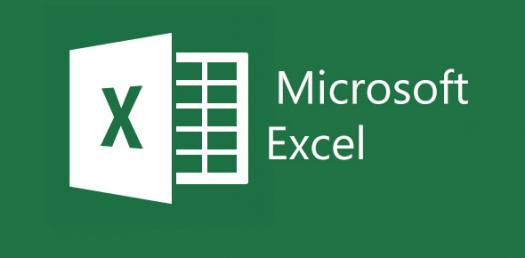# Test Your Basic Knowledge On MS Excel!

25 Questions | Total Attempts: 236SettingsDo you want to test your basic knowledge on excel sheet? Let's play this excel practice quiz and prove yourself!

Related Topics
• 1.
Labels are aligned at the ________ edge of the cell.
• A.

Left

• B.

Right

• C.

Top

• D.

Bottom

• 2.
A_______ is a group of cells that form a retangle on the screen.
• A.

Label

• B.

Workbook

• C.

Worksheet

• D.

Column

• E.

Range

• 3.
How do you know a range is active?
• A.

It is highlighted on the screen.

• B.

It has a marquee around it.

• C.

The data changes to Bold to let me know it's active

• D.

I'm psychic & I just Know!!

• 4.
An example of a  range address.
• A.

A1:B3

• B.

A1;B3

• C.

A1-B3

• D.

A1=B3

• E.

A1*B3

• 5.
On an Excel sheet the active cell is indicated by ____.
• A.

A dark wide border

• B.

A dotted border

• C.

• D.

None of the above

• 6.
Which formula can add the all the numeric values in a range of cells, ignoring those which are not numeric, and place the result in a different cell ?
• A.

Count

• B.

Average

• C.

Sum

• 7.
Is it possible to insert an image from a file into an Excel spreadsheet ?
• A.

Yes

• B.

No

• 8.
If cells: A1=90 A2=85 A3=80 A4=75 A5=75 What will be your formula if you are going to get the total?
• 9.
A fast way to add up this column of numbers is to click in the cell below the numbers and then:
• A.

Click Subtotals on the Data menu.

• B.

View the sum in the formula bar.

• C.

Click the AutoSum button on the Standard toolbar, then press ENTER.

• 10.
MS-EXCEL is based on ……….?
• A.

WINDOWS

• B.

DOS

• C.

UNIX

• D.

OS/2

• 11.
In EXCEL, you can sum a large range of data by simply selecting a tool button called …..?
• A.

AutoFill

• B.

Auto correct

• C.

Auto sum

• D.

Auto format

• 12.
How do you rearrange the data in ascending or descending order?
• A.

Data, Sort

• B.

Data, Form

• C.

Data, Table

• D.

Data Subtotals

• 13.
Which of the following is not a term of MS-Excel?
• A.

Cells

• B.

Rows

• C.

Columns

• D.

Document

• 14.
The first cell in EXCEL worksheet is labeled as
• A.

AA

• B.

A1

• C.

Aa

• D.

A0

• 15.
The cell range that starts in cell A1 and goes over to column J and down to row 15 is _____
• A.

A1-J15

• B.

A1.J15

• C.

A1;J15

• D.

A1:J15

• 16.
The cell reference for a range of cells that starts in cell B1 and goes over to column G and down to row 10 is
• A.

B1.G10

• B.

B1-G10

• C.

B1:G10

• D.

1:10:B:G

• 17.
The settings to wrap text in a cell and to merge cells are found in the format cells dialoge on the _______ tab
• A.

Number

• B.

Alignment

• C.

Font

• D.

Border

• 18.
Which of the folloiwing is a cell refernce?
• A.

C15

• B.

5C

• C.

CC

• D.

65,481

• 19.
If you have a value in cell K6, what do you have in the cell?
• A.

Letters

• B.

Words

• C.

Sentences

• D.

Numbers

• 20.
A cell reference is the number of a row then the letter of the column.
• A.

True

• B.

False

• 21.
A file in Excel is made up of 3 ______________ .
• A.

Rows

• B.

Columns

• C.

Worksheets

• D.

Workbooks

• 22.
In Microsoft Excel 2010, Where can you find the open, save, and print commands?
• A.

Home Ribbon

• B.

File Tab

• C.

Workbook Layout Button

• D.

Page Layout Button

• 23.
What do we call the boxes on a spreadsheet?
• A.

Formula bars

• B.

Grids

• C.

Home Boxes

• D.

Cells

• 24.
A _______ is a line of boxes going from left to right.
• A.

Row

• B.

Column

• C.

Formula String

• D.

• 25.
Which of the following formulas could you use to add several cells together?
• A.

=A1*B1*C1

• B.

A1+B1+C1=D1

• C.

=A1+B1+C1

• D.

A1*B1*C1